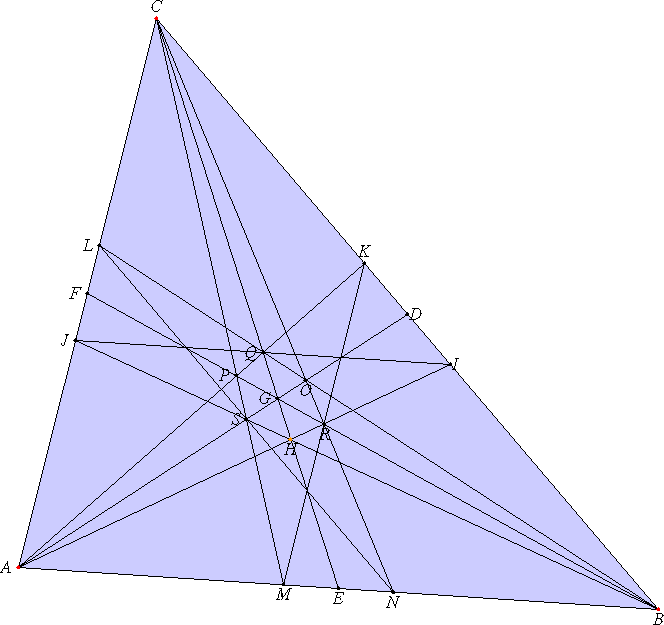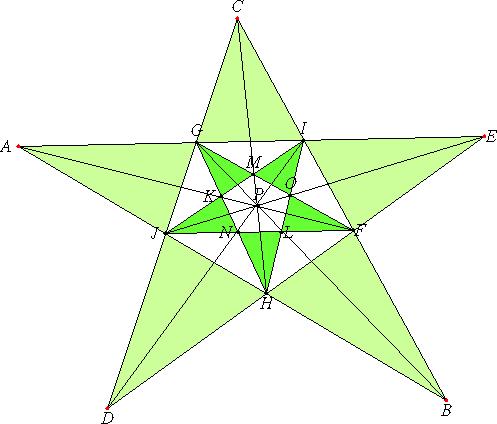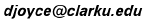### 16 points in 15 lines

Problem 868, Spear the Points, from Stan Wagon's "Problem of the Week" is this:
What is the greatest number of lines you can draw through 16 points so that each line contains exactly 4 points. You may place the 16 points anywhere in the plane you wish. (A line is doubly infinite.) [Source: Noam Elkies in Horizons, Sept. 1998]

(See below for more on Stan Wagon's POTW.)

I don't know the answer, but I can arrange the 16 points on 15 lines in the plane so that each line passes through exactly 4 points.

Take a triangle ABC. Place a point N on the side AB so that the ratio AN:NB is 1 less than the square root of 5. Put M on the same line but so that the ratio BM:MA has that ratio. Similarly place K and I on the side BC, and L and J on the side AC. Complete the diagram.(In this diagram, the point H is movable, but in actuality, it can only be placed in only one point on the line CG.)

Note that ten points each lie on three lines, and six points each lie on five lines.

#### Stan Wagon's solution

Stan's solution also gets 15 lines, but it's easier to find them.
Start with the five vertices of a regular star pentagon. Make another star pentagon using the five points inside the first one that are determined by intersections. This array has 15 points in it, counting all intersections. Add the center of the array.(In this figure, all five vertices of the pentagram are movable, but not all positions give proper intersection of the lines.)

#### The relation between these two figures

Note that with this solution, it is also the case that ten points each lie on three lines, and six points each lie on five lines. It may appear that these two configurations are different, but it is possible to move around the points on the pentagram in order to make the triangle in the first figure.

More prcisely, there's a projectivity of the plane which maps one figure to the other. The easiest way to see the correspondence is to start with a dodecahedron or an icosahedron. Consider the six lines through the centers of the opposite pairs of pentagonal faces of a dodecahedron (which are the six lines connecting the opposite vertices of an icosahedron), and the ten lines through the opposite vertices of the the dodecadron (which are the ten lines through the centers of the opposite paris of triangular faces of a dodecahedron.

Intersect these 16 lines with any plane and you get 16 points in that plane which lie on 15 lines. (The lines are intersections with that plane of planes determined by opposite pairs of edges of the original dodecahedron or icosahedron.) In particular, if the plane is parallel to a pentagonal face of the dodecahedron, then you get the pentagrammic figure; if it is parallel to a triangular face of the icosahedron, then you get the triangular figure.

Incidentally, if you take any 4 points a, b, c, and d in a plane (the projective plane), no three of which are collinear, then the rest of the 16 points are determined. That is, there are unique points e, f, 1, 2, ..., 10 so that

lines ab, cd, and ef intersect at 1
lines bc, de, and af intersect at 2
lines cd, ea, and bf intersect at 3
lines de, ab, and cf intersect at 4
lines ea, bc, and df intersect at 5

lines ac, be, and df intersect at 6
lines bd, ca, and ef intersect at 7
lines ce, db, and af intersect at 8
lines da, ec, and bf intersect at 9
lines eb, ac, and cf intersect at 10

I don't know if there is more than this one configuration that gives 15 lines. I also don't know if it's possible to get more than 15 lines. But there can't be any more than 20. Here's why. If any point lies on 6 lines, then you would need three more points on each for a total of 19 points, three more than we have. So, each point lies on at most 5 lines. If they all did, that would give 80 intersections of points and lines. But each line uses 4 of these intersections, so there can be at most 20 lines.

### Stan Wagon's Problems of the Week

The following statement is taken from the POTW-L mailing list.

The Problem of the Week from Stan Wagon at Macalester College in St. Paul, Minnesota wagon@macalester.edu. This Macalester tradition was started by the late Joe Konhauser in 1968 and has continued unbroken since then.

These problems are intended for our undergraduates. I do not necessarily wish to receive e-solutions unless:

(a) you have an interesting nonstandard approach to a solution;

(b) you have a variation or extension that might be worthy of dissemination; or

(c) you have information about the history of the problem. Of course, I encourage your problem suggestions.

#### Problem Archives:

1. There is an electronic archive of our Problems of Week at http://www.cs.duke.edu/~jeffe/wagon/ . But this is now being discontinued; a replacement based at Swarthmore College will be in place soon.

2. "Which Way Did the Bicycle Go?" by Joseph Konhauser, Dan Velleman, and Stan Wagon is available from the MAA. This book consists of 191 problems and solutions selected from the Problems of the Week at Macalester College from 1968 to 1995. Contact: 800-331-1622 or http://www.maa.org

#### POW Subscriptions:

One can subscribe to the list by sending a message containing only the line:
```    SUBSCRIBE POTW-L
```
to mailserv@macalester.edu.

To simply unsubscribe, send a message to << mailserv@macalester.edu >> from the account to be unsubscribed and containing the body:

```    UNSUBSCRIBE POTW-L
```